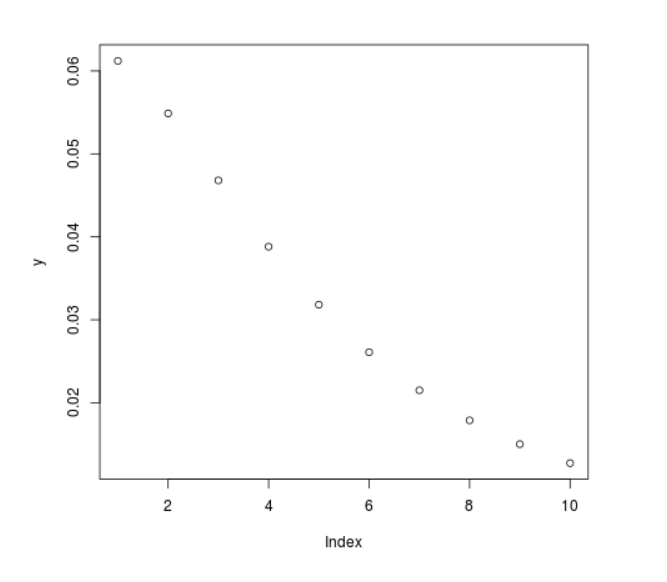# Compute Cauchy Density in R Programming – dcauchy() Function

`dcauchy()` function in R Language is used to calculate the cauchy density. It also creates a density plot of cauchy distribution.

Syntax: dcauchy(vec, scale)

Parameters:
vec: x-values for cauchy function
scale: Scale for plotting

Example 1:

 `# R Program to compute cauchy density ` ` `  `# Creating vector for x-values ` `x <``-` `seq(``1``, ``10``, by ``=` `1``) ` ` `  `# Apply dcauchy() Function ` `y <``-` `dcauchy(x, scale ``=` `5``) ` `y `

Output:

```  0.06121344 0.05488101 0.04681028 0.03881828 0.03183099 0.02609097
 0.02150742 0.01788258 0.01501462 0.01273240
```

Example 2:

 `# R Program to compute cauchy density ` ` `  `# Creating vector for x-values ` `x <``-` `seq(``1``, ``10``, by ``=` `1``) ` ` `  `# Apply dcauchy() Function ` `y <``-` `dcauchy(x, scale ``=` `5``) ` ` `  `# Plotting a graph ` `plot(y) `

Output:My Personal Notes arrow_drop_upCheck out this Author's contributed articles.

If you like GeeksforGeeks and would like to contribute, you can also write an article using contribute.geeksforgeeks.org or mail your article to contribute@geeksforgeeks.org. See your article appearing on the GeeksforGeeks main page and help other Geeks.

Please Improve this article if you find anything incorrect by clicking on the "Improve Article" button below.

Article Tags :

Be the First to upvote.

Please write to us at contribute@geeksforgeeks.org to report any issue with the above content.